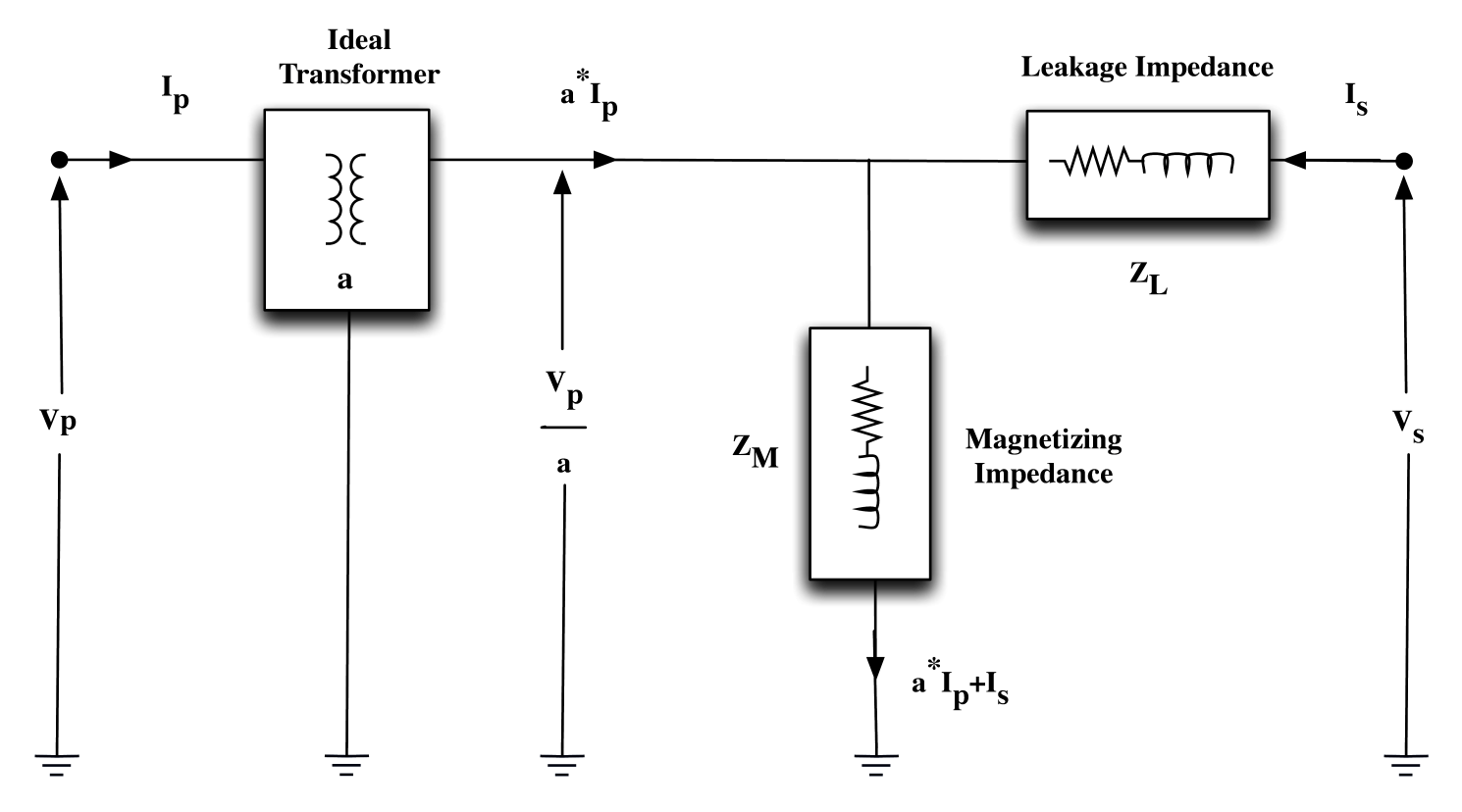# 1 Transformer Equivalent Circuit

The equivalent circuit of a two winding transformer consists of an ideal transformer, a leakage impedance ZL, and a magnetizing impedance ZM. An ideal transformer is a lossless entity categorized by a complex voltage ratio a, i.e.

 $\mathbf{a=\frac{V_{p}}{V_{s}}}$ (1)

where

Vp is the transformer’s primary voltage.

Vs is the transformer’s secondary voltage.

Since the ideal transformer has no losses, it must be true that

 $\mathbf{S_{p}=S_{s}}$ (2)

or

 $\mathbf{V_{p}{I^{\star}_{p}}=V_{s}{I^{\star}_{s}}}$ (3)

Solving for the secondary current

 $\displaystyle\mathbf{I^{\star}_{s}}$ $\displaystyle=\mathbf{\left(\frac{V_{p}}{V_{s}}\right){I^{\star}}_{p}}$ (4) $\displaystyle\mathbf{I_{s}}$ $\displaystyle=\mathbf{{\left(\frac{V_{p}}{V_{s}}\right)}^{\star}I_{p}}$ (5)

Substituting Equation 1 reveals the relationship between the primary and secondary current of an ideal transformer

 $\mathbf{I_{s}}=\mathbf{a^{\star}I_{p}}$ (6)

where

Ip is current into the transformer’s primary.

Is is current out of the transformer’s secondary.

a is the transformer’s voltage transformation ratio.

A transformer’s magnetizing impedance is a shunt element associated with its excitation current, i.e. the “no load” current in the primary windings. The real component of the magnetizing impedance reflects the core losses of the transformer. Its reactive component describes the mmf required to overcome the magnetic reluctance of the core.

A transformer’s leakage impedance is a series element that reflects imperfections in the windings. The real component of the leakage impedance is the resistance of the windings. Its reactive component is due to leakage flux, i.e. flux in the magnetic circuit that does not contribute to coupling the windings.

Figure 1 depicts a transformer’s equivalent circuit with impedances referred to the secondary.

Figure 1: Transformer Equivalent Circuit Diagram